# PAS 13: what ratings mean

Understand how ratings are calculated and what they mean.

KE = ½m x (vsinΘ)²

## How impact ratings are calculated

To help you understand what an impact rating means we will break down all the component parts of the calculation, enabling you to be fully informed and ask the right questions to guarantee the barriers you select are up to the job.

This will also enable you to calculate the maximum force a barrier will need to withstand based on the vehicles used within your facility and the layout.

The equation to calculate the amount of energy transferred is KE = ½m x (vsinΘ)²

where:​

• KE is the kinetic energy (in joules (J);​
• m is the mass of the vehicle (in kg);​
• v is the velocity of the vehicle (in m/s);​
• Θ is the impact angle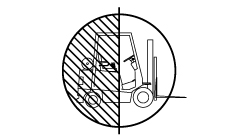## Mass

The mass of a vehicle is measured in kg, but you will also need to factor in the maximum load any vehicle may be carrying.​

​For this example we will use a 4,000kg vehicle carrying 600kg. This means the mass (m) = 4,600kg.​

​Let’s input this into the equation:​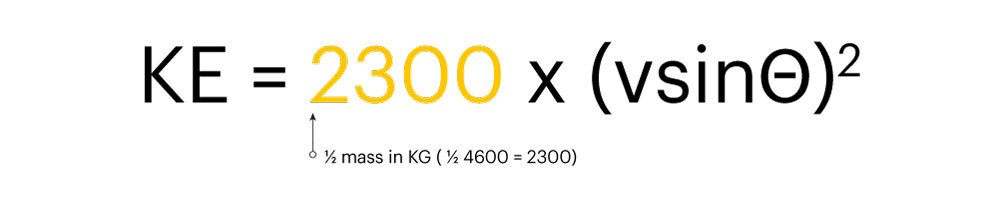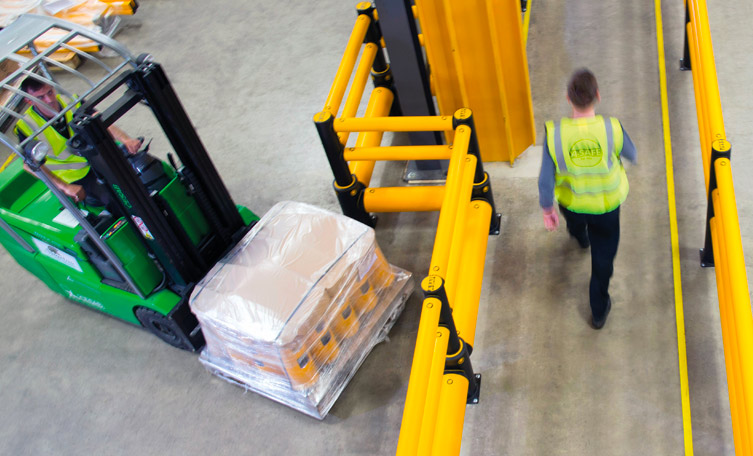## Velocity

Once you have calculated the mass of the vehicle, you will need to determine its velocity (v). This is measured in metres per second (m/s). To calculate m/s, you must first determine the kilometres per hour (km/h).​

If you work in miles per hour (mph), you will need to multiply the mph figure by 1.61 to calculate the km/h figure. Once you have the km/h figure, you will need to divide it by 3.6 to calculate the m/s figure. ​

For this example, the vehicle is travelling at 5mph. This means that km/h = 5 x 1.61 = 8.05km/h. Therefore, the velocity (v) = 8.05 ÷ 3.6 = 2.236.​

Let’s input this into our equation:​​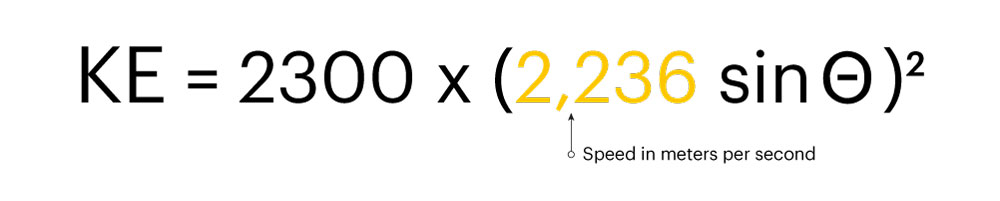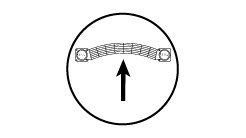## Impact angle

The impact angle will depend on a number of variables including the dimensions of the vehicle, the vehicle’s turning circle and the width of the vehicle route.​

​Impact angles affect the total amount of kinetic energy that is transferred during impact. The shallower or more narrow the angle, the less energy that is transferred. At 90 degrees, all of the energy is transferred during impact.​

​To calculate this, you need the sine of the angle. Please see below the figures for common angles that may be used for the sinΘ calculation:​

• Sin90 ° = 1​
• Sin67.5 ° = 0.924​
• Sin45 ° = 0.707​
• Sin22.5 ° = 0.383​
• Sin10 ° = 0.1736​

​For our example, we are using an impact angle of 90°. This means 'Θ’ = 1.​

​Let’s input this into our equation:​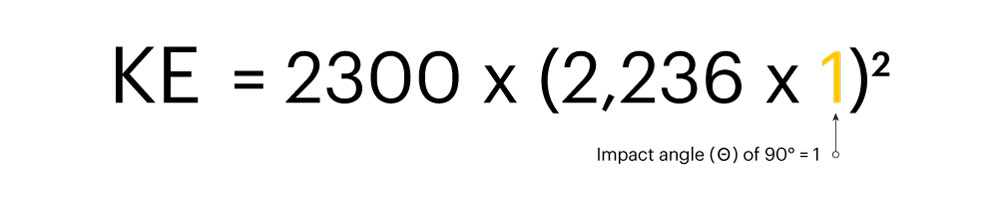## Kinetic energy

Kinetic energy is measured in Joules which is a unit used to measure energy or force. We rate all of our products to include a safety margin for your protection​.

​The outcome of our calculation means a vehicle with a total weight of 4,600kg travelling at 5mph and impacting a barrier at 90 degrees will transfer 11,499 Joules of energy to the barrier. ​

​KE = 2,300 x (2.236 x 1)²

KE = 2,300 x (2.236)²

KE = 2,300 x 4.999​

KE = 11,499 Joules

​This means that the safety barrier installed to protect against this type of impact would need to be able to withstand a minimum of 11,499 Joules of energy.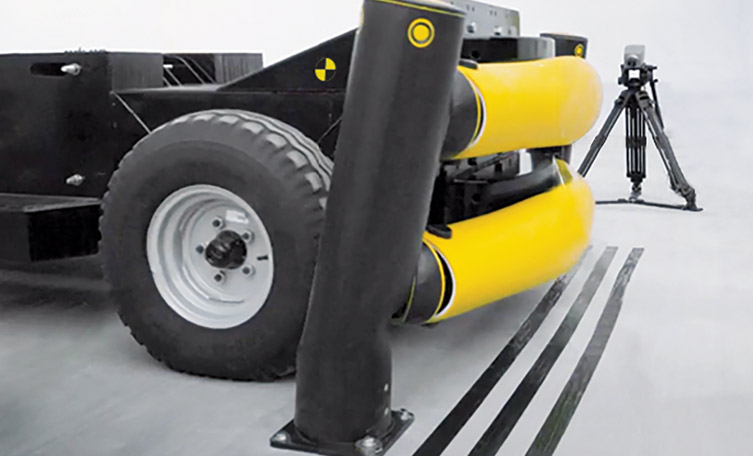PAS 13 Safety Barrier Testing | Standardising Impact Ratings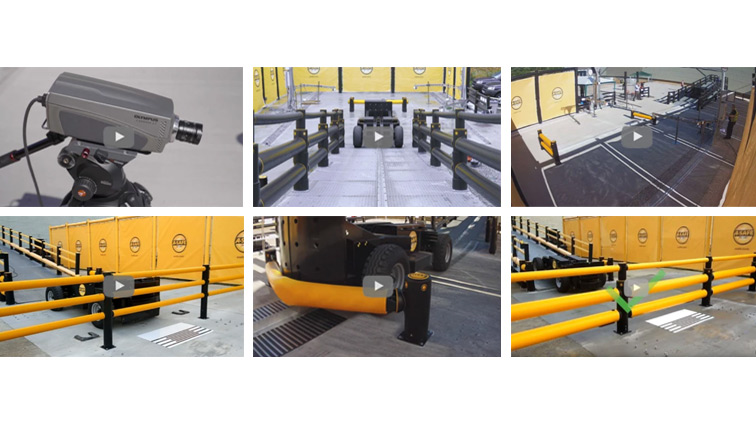See exactly how we set up and test our barriers inline with PAS 13 with our PAS 13 code of practice video series.

Know your equipment What makes a compliant vehicle? How should an impact area be prepared? The steps to follow What does a full test look like? Recording and reporting your results Barrier testing: Pass or fail